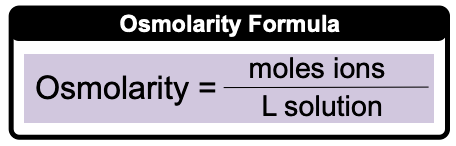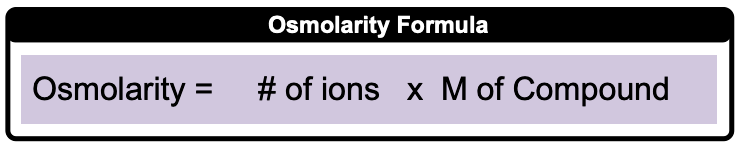Clutch Prep is now a part of Pearson
Ch.4 - Chemical Quantities & Aqueous ReactionsWorksheetSee all chapters

# Osmolarity

See all sections
Sections
Solutions
Molarity
Osmolarity
Dilutions
Solubility Rules
Electrolytes
Molecular Equations
Gas Evolution Equations
Solution Stoichiometry
Complete Ionic Equations
Calculate Oxidation Numbers
Redox Reactions
Balancing Redox Reactions: Acidic Solutions
Balancing Redox Reactions: Basic Solutions
Activity Series

Osmolarity (ionic molarity) represents the number of moles of ions per liter of solution.

###### Osmolarity

Concept #1: Method 1: Direct Calculation of Osmolarity

Direct Calculation of Osmolarity:Example #1: Calculate the molarity of chloride ions when dissolving 58.1 g AlCl3 in enough water to make 500 mL of solution.

Concept #2: Method 2: Osmolarity from Molarity

Osmolarity from Molarity of compound:Example #2: What is the concentration of hydroxide ions in a 0.350 M solution of gallium hydroxide, Ga(OH)3?

Example #3: How many moles of Ca2+ ions are in 0.120 L of 0.450 M Ca3(PO4)2 solution?

Using Molarity of compounds and L of solutions, moles of ions can be calculated.

Practice: Which of the following solutions will have the highest concentration of bromide ions?

Practice: How many milligrams of nitride ions are required to prepare 820 mL of 0.330 M Ba3N2 solution?

Practice: How many bromide ions are present in 65.5 mL of 0.210 M GaBrsolution?